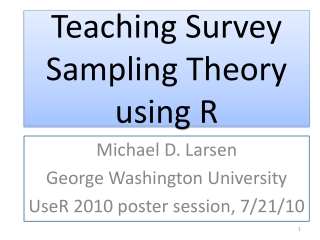DownloadDownload PresentationTeaching Survey Sampling Theory using R

# Teaching Survey Sampling Theory using R

Télécharger la présentation## Teaching Survey Sampling Theory using R

- - - - - - - - - - - - - - - - - - - - - - - - - - - E N D - - - - - - - - - - - - - - - - - - - - - - - - - - -
##### Presentation Transcript

1. Teaching Survey Sampling Theory using R Michael D. Larsen George Washington University UseR 2010 poster session, 7/21/10

2. Uses of R in the course • Data analysis; exploring data • Programming complex formulas • Simulation of properties of estimators • Make estimation easier so one can think about concepts

3. Exploring data • Examine means, sizes of clusters: more variability increases variance • Examine means, sizes across strata: more variability decreases variance • Examine skewness of variables: extreme skewness in population can lead to unrealistic sample-based estimates

4. Exploring Data: Tools • Side-by-side boxplots • boxplot(split(senic\$nurses, senic[,c("region","medical")]), xlab="four regions in U.S.; two hospital types", ylab="# nurses", main="113 hospitals in U.S.") • Histograms • Numerical summaries • Correlations; regression • sapply for lists created using ‘split’ command

5. Comparing two factors for stratification potential

6. Programming complex formulas • Checks understanding of formulas • Helps memorization of formulas • Next page: two-stage cluster sample estimator for total and variance of total

7. Simulation • Using functions, one can contrast complex estimation methods in terms of bias, variance and MSE • Simulating 1,000 samples and plotting results gives different impression than mathematical result; Impact of skewness and outliers is more transparent

8. Ease of use • Make estimation easier so one can think about concepts; Possible to focus on contrasts and more variables • Students can do more ambitious projects and handle ‘real’ data

9. Ease of use example For a given budget and population, what is the advantage of more clusters with smaller sample sizes versus fewer clusters with bigger sample sizes? • Compute variances under three scenarios. • Take 10,000 samples under three different scenarios and compute variance of estimates. • Apply to three different variables. Write a summary.

10. Suggestions, part 1 • Consistent syntax across survey designs • Ease of use: be clear on what time of variables are needed – factor, numeric, etc. • More examples with more numbers that can be replicated

11. Suggestions, part 2 • Recover formula – not only R syntax but also an estimation formula – when run command • More details in context when errors occur: • Your sample sizes for clusters (ni) exceed your population sizes for clusters (Ni). • Only one primary sampling unit (defined by psu) is available for some clusters • Include writing projects based on data analysis